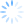Can CDXZipStream be used in other applications besides Excel, such as Microsoft Access, Word, Powerpoint, or Sharepoint?  Yes it can! Using some simple Visual Basic code, it is fairly easy to “call” CDXZipStream and get the data you need from the application of your choice.  In this way you can obtain CDXZipstream data without using custom function formulas in worksheet cells. Other advantages to this approach is that it can be faster for large data sets, and it eliminates delays associated with Excel or any other application recalculating worksheet formulas.  We use this approach in our free, downloadable templates, which are especially quick and efficient for processing long lists of data.

In a previous post we reviewed how to use VBA, or Visual Basic for (Office) Applications, to access CDXZipStream custom functions CDXZipCode, CDXLocateMP, CDXDistance2WP, and CDXRouteMP.   So let’s finish the task for all the other available functions:

To start, open Excel and press ALT-F11 to access the Visual Basic for Applications (VBA) editor.   From the Insert menu of the editor, select Module, and in the new module window that opens you can now apply the following code for each function:

CDXFindZip – finds the ZIP code(s) for a given city and state.

In the first line of code we use the createobject statement to connect to CDXZipStream:

Then simply ask for the data (that is assigned here to a variable called ZIP):

ZIP = CDXFindZip("Abington","Massachusetts")

The code can be generalized to obtain data for a long list city and state pairs.  In Microsoft Excel, for example, just loop through the worksheet range containing the city and state data.  Here is the basic code you can copy to a module, and modify as needed:

Sub FindZIP

Dim City as string

Dim State as string

Dim Zip as string

Dim N as integer

For N = 1 to 100

City = Sheets(1).Range(“A” & N & “”)   ‘Get each city from the worksheet list

State = Sheets(1).Range(“B” & N & “”)   ‘Get each state from the worksheet list

Sheets(1).Range(“C” & N & “”) = Zip    ‘Place the returned data in the worksheet next to its corresponding zip code

Next N

End Sub

All other functions follow a similar format:

CDXDistance – finds the straight-line distance between two ZIP codes

Dim Zip1 As String

Dim Zip2 As String

Dim Distance As String

The equation above obtains the distance in miles.  Use the following formats to obtain distance in kilometers and nautical miles, respectively:

CDXRadius – finds all ZIP codes within a radius distance of a target ZIP code

Dim Zip As String   (The target Zip code, the center of the radius area)

Dim Output As Integer  (Specifies whether the returned data are in a single string or array)

Dim Unit As String (Specifies the returned distance data as miles, kilometers, or nautical miles)

Dim Database As Integer (Specifies use of U.S. Zip codes, or Canadian postal codes, or both)

Dim Zip_list as variant  (The results returned as a string or array)

If the data is returned as an array, it will also be necessary to extract the data to individual cells in the worksheet, as follows:

For I = 0 To UBound(Zip_list, 1)' Retrieve data from array

Sheets(1).Cells(I + 11, N) = Zip_list (I, 0)

Sheets(1).Cells(I + 11, N + 1) = Zip_list (I, 1)

Next I

CDXClosest – finds the first, second, or third closest ZIP code from a target ZIP code

Since this function requires a range as input, the code is slightly different.

Dim Target As String  (The target Zip code)

Dim Output As Integer (The type of returned data, i.e. first closest, second closest, etc.)

Dim ZipArray As Variant  (The range of Zip codes that will be searched)

Dim Result As String

To create the ZipArray, loop through the range that contains the data, in this case, in rows 1 through 10:

ReDim ZipArray (1 to 10)

For N = 1 To 10

ZipArray(N) = Sheets(1).Range("A" & N & "")

End If

Next N

CDXZiplist – finds all ZIP codes for a given city, county, or state.

Dim Output As Integer (Specifies whether the returned data are in a single string or array)

Dim Postal_option As Integer (Specifies whether all data, or only Zip or postal data, is returned)

Dim State As String

Dim County As String

Dim City As String

Dim Zip_list as variant

(Note that city and county data are optional.  For example, specifying only state data would provide all the Zip codes for the specified state.)